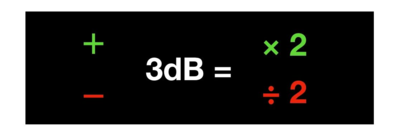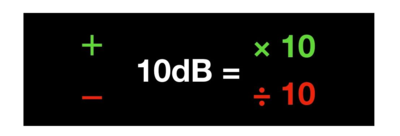# 如何快速口算转换dBm与W？一文彻底了解清楚

Tx是发射（ Transmits ）的简称。无线电波的发射功率是指在给定频段范围内的能量，通常有两种衡量或测量标准：

dBm = 10 x log[ 功率/mW]
mW = 10 [ 增益 dBm / 10 dBm]

dBm 定义是分贝毫瓦。是一个表示功率绝对值的值（也可以认为是以1mW功率为基准的一个比值）,计算公式为：dBm = 10log ( P /1 mw)。1、＋3dB，功率乘以2；－3dB，功率除以22、＋10dB，功率乘以10；－10dB，功率除以10100mW + 3dB = 100×2 = 200（mW）

100mW + 10dB = 100 × 10 = 1000（mW）

100mW + 7dB = 100mW + （10dB - 3dB）
=100 × 10 ÷ 2
= 500（mW）

44dBm = 30dBm + 10dB + 10dB－3dB－3dB
=1W×10×10÷ 2 ÷ 2
= 25W

32dBm = 30dBm + 3dB + 3dB + 3dB + 3dB－10dB
= 1W×2×2×2×2÷10
= 1.6W

+1dB = +10dB－3dB－3dB－3dB
= X×10×1/2×1/2×1/2
= X×1.25

+2dB = －10dB+3dB+3dB+3dB+3dB
= X×0.1×2×2×2×2
= X×1.6

－1dB = －10dB+3dB+3dB+3dB
= X×0.1×2×2×2
= X×0.8

－2dB = －3dB+1dB
= X×1/2×1.25
= X×0.625

51dBm = 30dBm+10dB+10dB+1dB
= 1W×10×10×1.25
= 125W

38dBm = 30dBm + 10dB－2dB
= 1W×10×0.625
= 6.25W15975594909

0757-85687501

-------------------------------------------------------------------------------------------------------------------------------------